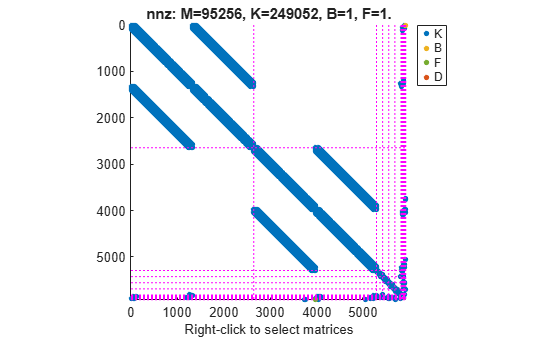Main Content

# Rigid Assembly of Model Components

This example shows how to specify rigid physical coupling between the components of a structural model. Consider a structure that consists of two square plates connected with pillars at each vertex as depicted in the figure below. The lower plate is connected rigidly to the ground while the pillars are connected rigidly to each vertex of the square plates.

For more information on sparse models, see Sparse Model Basics.`platePillarModel.mat` contains the sparse matrices for pillar and plate model. Load the finite element model matrices contained in `platePillarModel.mat` and create the sparse second-order state-space model representing the above system.

```load('platePillarModel.mat') sys = ... mechss(M1,[],K1,B1,F1,'Name','Plate1') + ... mechss(M2,[],K2,B2,F2,'Name','Plate2') + ... mechss(Mp,[],Kp,Bp,Fp,'Name','Pillar3') + ... mechss(Mp,[],Kp,Bp,Fp,'Name','Pillar4') + ... mechss(Mp,[],Kp,Bp,Fp,'Name','Pillar5') + ... mechss(Mp,[],Kp,Bp,Fp,'Name','Pillar6')```
```Sparse continuous-time second-order model with 1 outputs, 1 inputs, and 5820 nodes. Use "spy" and "showStateInfo" to inspect model structure. Type "properties('mechss')" for a list of model properties. Type "help mechssOptions" for available solver options for this model. ```

The resultant model `sys` has 5820 nodes where every node has two degrees of freedom (DoF) which are $\left[q,\underset{}{\overset{˙}{q}}\right]$.

Use `showStateInfo` to examine the components of the `mechss` model object.

`showStateInfo(sys)`
```The state groups are: Type Name Size ---------------------------- Component Plate1 2646 Component Plate2 2646 Component Pillar3 132 Component Pillar4 132 Component Pillar5 132 Component Pillar6 132 ```

The named components are listed in the command window with their respective node sizes.

Now, load the interfaced node index data from `nodeData.mat` and use `interface` to create the physical connections between the two plates and the four pillars. `nodes` is a `6x7` cell array where the first two rows contain node index data for the first and second plate while the remaining four rows contain index data for the four pillars.

`load('nodeData.mat','nodes')`

Now, specify rigid couplings between the plates and the pillars.

```for i=3:6 sys = interface(sys,"Plate1",nodes{1,i},"Pillar"+i,nodes{i,1}); sys = interface(sys,"Plate2",nodes{2,i},"Pillar"+i,nodes{i,2}); end```

Specify rigid connection between the bottom plate and the ground.

`sysCon = interface(sys,"Plate2",nodes{2,7})`
```Sparse continuous-time second-order model with 1 outputs, 1 inputs, and 5922 nodes. Use "spy" and "showStateInfo" to inspect model structure. Type "properties('mechss')" for a list of model properties. Type "help mechssOptions" for available solver options for this model. ```

Notice that the model now contains 5922 nodes. The extra nodes are a result of the specific rigid interfaces.

`interface` uses 'dual assembly' formulation to connect the components. In the concept of dual assembly, the global set of degrees of freedom (DoFs) $\mathit{q}$ is retained and the physical coupling is expressed as consistency and equilibrium constraints at the interface. For rigid connections, these constraints are of the form:

`$Bq=0,\phantom{\rule{0.2777777777777778em}{0ex}}\phantom{\rule{0.2777777777777778em}{0ex}}\phantom{\rule{0.2777777777777778em}{0ex}}\phantom{\rule{0.2777777777777778em}{0ex}}\phantom{\rule{0.2777777777777778em}{0ex}}\phantom{\rule{0.2777777777777778em}{0ex}}\phantom{\rule{0.2777777777777778em}{0ex}}\phantom{\rule{0.2777777777777778em}{0ex}}\phantom{\rule{0.2777777777777778em}{0ex}}\phantom{\rule{0.2777777777777778em}{0ex}}g=-{B}^{T}\lambda$`

where $\mathit{g}$ is the vector of internal forces at the interface, and the matrix $\mathit{B}$ is permutable to $\left[\mathit{I}\text{\hspace{0.17em}}\text{\hspace{0.17em}}-\mathit{I}\right]$. For a pair of matching nodes with indices ${\mathit{i}}_{\mathit{i}}$, ${\mathit{i}}_{2}$ where ${\mathit{i}}_{\mathit{i}}$ selects a node in the first component while ${\mathit{i}}_{2}$ selects the matching node in the second component, $Bq=0$ enforces consistency of displacements:

`$q\left({i}_{1}\right)=q\left({i}_{2}\right)$`

while $g=-{B}^{T}\lambda$ enforces equilibrium of the internal forces g at the interface:

$g\left({i}_{1}\right)+g\left({i}_{2}\right)=0$.

Combining these constrains with the uncoupled equations $M\underset{}{\overset{¨}{q}}+C\underset{}{\overset{˙}{q}}+Kq=f+g$ leads to the following dual assembly model for the coupled system:

`$\left[\begin{array}{cc}M& 0\\ 0& 0\end{array}\right]\left[\begin{array}{c}\underset{}{\overset{¨}{q}}\\ \lambda \end{array}\right]+\left[\begin{array}{cc}C& 0\\ 0& 0\end{array}\right]\left[\begin{array}{c}\underset{}{\overset{˙}{q}}\\ \lambda \end{array}\right]+\left[\begin{array}{cc}K& {B}^{T}\\ B& 0\end{array}\right]\left[\begin{array}{c}q\\ \lambda \end{array}\right]=\left[\begin{array}{c}f\\ 0\end{array}\right]$`

For more information, see `interface`.

Use `showStateInfo` to confirm the physical connections.

`showStateInfo(sysCon)`
```The state groups are: Type Name Size ----------------------------------- Component Plate1 2646 Component Plate2 2646 Component Pillar3 132 Component Pillar4 132 Component Pillar5 132 Component Pillar6 132 Interface Plate1-Pillar3 12 Interface Plate2-Pillar3 12 Interface Plate1-Pillar4 12 Interface Plate2-Pillar4 12 Interface Plate1-Pillar5 12 Interface Plate2-Pillar5 12 Interface Plate1-Pillar6 12 Interface Plate2-Pillar6 12 Interface Plate2-Ground 6 ```

You can use `spy` to visualize the sparse matrices in the final model. Choose between the matrices to be displayed using the display menu that can be accessed by right-clicking on the plot.

`spy(sysCon)`### Acknowledgements

The data set for this example was provided by Victor Dolk from ASML.

## Support

#### Learn how to automatically tune PID controller gains

Download code examples